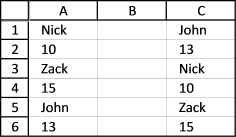Author: Oscar Cronquist Article last updated on July 15, 2017

I showed you in an earlier post how to sort text by number using a formula, it was a question from Denisa. The first thing that comes to mind would be to rearrange the values and then apply a filter or an excel defined table to be able to sort the the names by value.

In other words, names in one column and numbers in another column. But I didn't, I built a formula, shown in row 3 (A3:F3), it was an interesting challenge that I could not resist.15 is the largest number and Zack is the corresponding name. 13 is the second largest number and John is the name next to it. 10 is the smallest number and the adjacent name is Nick. Check out the post if you want to know more.

In this post I will show you a formula that sorts numbers by text, a little bit different than the previous post mentioned above. The values are in column A and the formula will sort the names alphabetically and also return the corresponding number in column C, see picture below.The formula is in column C.

=INDEX(\$A\$1:\$A\$6, MATCH(SMALL(IF(ISTEXT(\$A\$1:\$A\$6), COUNTIF(\$A\$1:\$A\$6, "<"&\$A\$1:\$A\$6), ""), ROUND(ROW(A1)*0.5, 0)), IF(ISTEXT(\$A\$1:\$A\$6), COUNTIF(\$A\$1:\$A\$6, "<"&\$A\$1:\$A\$6), ""), 0)+MOD(ROW(A2), 2))

You must enter this formula as an array formula. Here are the steps:

1. Copy and paste the formula in cell C1
2. Press and hold CTRL + SHIFT simultaneously
3. Press Enter once
4. Release all keys.

The formula is now surrounded by curly brackets, like this {=formula} if you did it right. Check your formula bar and make sure you have the curly brackets.

Then copy cell C1 and paste to cells beneath.

### Explaining the formula

The calculations below are for cell C1.

Sort data alphabetically

COUNTIF(\$A\$1:\$A\$6, "<"&\$A\$1:\$A\$6)

becomes

COUNTIF({"Nick";10;"Zack";15;"John";13}, "<"&{"Nick";10;"Zack";15;"John";13})

and returns {1; 0; 2; 2; 0; 1}

The COUNTIF function compares each value in the second argument with values in the first argument and labels them with numbers depending on their position in a sorted list. The magic is done by this code "<"& in the second argument. It appends a less than sign to each value in the second argument.

Filter text values

IF(ISTEXT(\$A\$1:\$A\$6), COUNTIF(\$A\$1:\$A\$6, "<"&\$A\$1:\$A\$6), "")

becomes

IF({TRUE;FALSE;TRUE;FALSE;TRUE;FALSE}, {1; 0; 2; 2; 0; 1}, "")

and returns {1;"";2;"";0;""}

We want to sort text values only, the IF and ISTEXT functions check if a value is a text value.

Find n-th the smallest number in array

SMALL(IF(ISTEXT(\$A\$1:\$A\$6), COUNTIF(\$A\$1:\$A\$6, "<"&\$A\$1:\$A\$6), ""), ROUND(ROW(A1)*0.5, 0))

becomes

SMALL({1;"";2;"";0;""}, ROUND(ROW(A1)*0.5, 0))

becomes

SMALL({1;"";2;"";0;""}, ROUND(0.5, 0))

becomes

SMALL({1;"";2;"";0;""}, 1)

and returns 0.

This part of the formula ROUND(ROW(A1)*0.5, 0) requires explanation, it returns a value depending on the relative cell reference A1. It changes as you copy the formula downwards. In cell C1 ROUND(ROW(A1)*0.5, 0) returns 1, C2 returns 1, C3 returns 2, C4 returns 2, C5 returns 3 and C6 returns 3. This makes it possible to get both the number and text from column A using the INDEX function, I will explain that later.

Find the position in the array

MATCH(SMALL(IF(ISTEXT(\$A\$1:\$A\$6), COUNTIF(\$A\$1:\$A\$6, "<"&\$A\$1:\$A\$6), ""), ROUND(ROW(A1)*0.5, 0)), IF(ISTEXT(\$A\$1:\$A\$6), COUNTIF(\$A\$1:\$A\$6, "<"&\$A\$1:\$A\$6), ""), 0)

becomes

MATCH(0, IF(ISTEXT(\$A\$1:\$A\$6), COUNTIF(\$A\$1:\$A\$6, "<"&\$A\$1:\$A\$6), ""), 0)

becomes

MATCH(0, {1;"";2;"";0;""}, 0)

and returns 5.

Return values from column A

INDEX(\$A\$1:\$A\$6, MATCH(SMALL(IF(ISTEXT(\$A\$1:\$A\$6), COUNTIF(\$A\$1:\$A\$6, "<"&\$A\$1:\$A\$6), ""), ROUND(ROW(A1)*0.5, 0)), IF(ISTEXT(\$A\$1:\$A\$6), COUNTIF(\$A\$1:\$A\$6, "<"&\$A\$1:\$A\$6), ""), 0)+MOD(ROW(A2), 2))

becomes

INDEX(\$A\$1:\$A\$6, 5+MOD(ROW(A2), 2))

becomes

INDEX(\$A\$1:\$A\$6, 5+0)

and returns "John" from cell A5.

As you copy the formula and paste it to cells below, this part changes MOD(ROW(A2), 2). There is a relative cell reference here also, MOD(ROW(A2), 2) returns 0 in cell C1, 1 in cell C2. This makes it possible to also fetch the corresponding value.